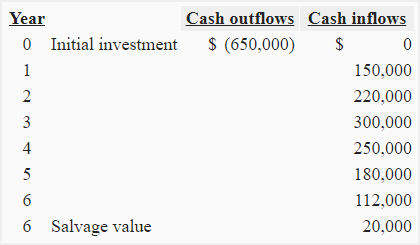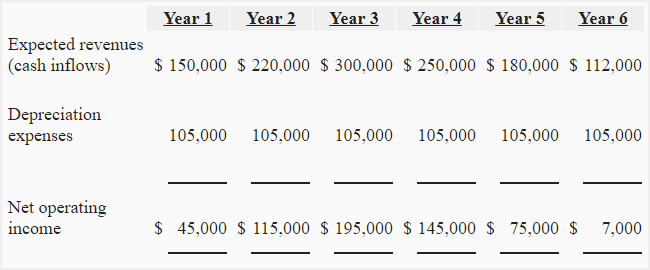# Exercise-13 (Accounting rate of return – uneven cash flows)

A project of \$650,000 is expected to generate the following cash flows over its useful life:The project does not require any cash expenses. Depreciation is to be provided using straight line method. According to accounting policies of the company, the salvage value is treated as the reduction in depreciable basis.

Required: Compute accounting rate of return from the above information.

## Solution:

### Step 1: Computation of annual depreciation expenses:

(Cost – salvage value)/life of the asset

(\$650,000 – \$20,000)/6

\$10,5000

### Step 2: Computation of average incremental annual income:Average income = (45,000 + 115,000 + 195,000 + 145,000 + 75,000 + 7,000)/6

= \$97,000

### Step 3: Computation of accounting rate of return:

#### If initial investment is used as denominator:

Accounting rate of return = Incremental accounting income/Initial investment

= \$97,000 / \$650,000

= 14.92%

#### If average investment is used as denominator:

Accounting rate of return = Incremental accounting income/Average investment

= \$97,000 / \$335,000*

= 28.96%

*Average investment in project:
(\$650,000 + \$20,000)/2
= \$335,000

Show your love for us by sharing our contents.
A D V E R T I S E M E N T